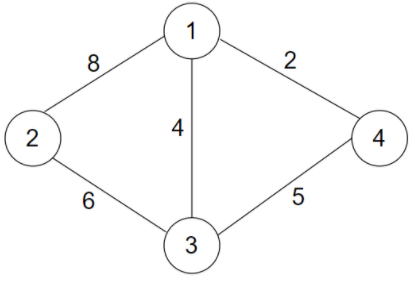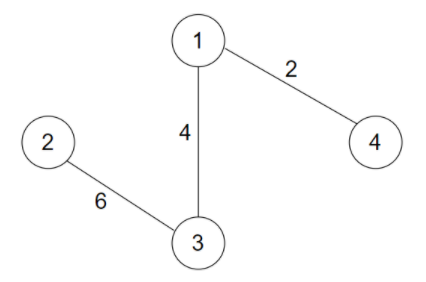New update is available. Click here to update.

# Minimum Travel Cost

Last Updated: 23 Dec, 2020
Difficulty: Moderate

## PROBLEM STATEMENT

#### Ninjaland is a country having 'N' states numbered from 1 to 'N'. These 'N' states are connected by 'M' bidirectional roads. Each road connects to different states and has some cost to travel from one state to another. Now, the chief wants you to select 'N' - 1 roads in such a way that the tourist bus can travel to every state at least once at minimum 'COST'.

##### For example :
``````Consider a country having 4 states numbered from 1 to 4. These 4 states are connected by 5 bidirectional roads given as :
1 --- 2 with cost = 8
2 --- 3 with cost = 6
3 --- 4 with cost = 5
1 --- 4 with cost = 2
1 --- 3 with cost = 4

The map of the country can be represented as:
````````````Now, the best way to choose 3 roads is:
````````````The cost of travelling from any state to all other states is  2 + 4 + 6 i.e. 12.
``````
##### Input format :
``````The first line contains an integer 'T' denoting the number of test cases or queries to be run.

The first line of each test case or query contains two space-separated integers 'N' and ‘M’ representing the number of states and number of roads in the country, respectively.

The next 'N' lines of every test case contain three single space-separated integers ‘A’, ‘B’ and ‘C’, representing a road between the states 'A' and 'B' and 'C' denoting the cost of travelling them.
``````
##### Output format:
``````For each test case, print 'N' - 1 lines each containing 3 space separated integers 'A', 'B' and 'C' representing the road you have chosen and the cost to traverse that road.
``````
##### Note:
``````You do not need to print anything, it has already been taken care of. Just implement the given function.
``````
##### Constraints:
``````1 <= 'T' <= 10
1 <= 'N' <= 1000
'N' - 1 <= 'M' <= 2000
1 <= 'C' <= 10^6

Time limit: 1 sec
``````## Approach 1

The idea is to create a graph of the country with states as its vertices and roads as edges.

For creating a graph we will use an adjacency matrix representation of graph Cost with Cost[i][j] representing the cost of travelling from state i to state j and vice versa, state j to i.

The very first approach to solve this problem is using Prim’s algorithm, which is a greedy algorithm.

The idea behind Prim’s algorithm is simple, a spanning tree means all states must be connected.

• We bifurcate the states in 2 groups:
• The first group contains the states already included in the MST.
• The other group contains the states not yet included.
• At every step, it considers all the roads that connect the two groups and picks the minimum weight road from these roads or minimum weight state from the second group.
• After picking the road, it adds the state of the second group into the first group ( MST group).

Algorithm:

1. Firstly, create an adjacency matrix of the given roads and costs.
2. Create a visited array of size N that keeps track of states already included in MST. Initially mark all states as unvisited.
3. Create a weight array of size N, to assign a weight value to all states in the input graph. Initialize all weight values as INFINITE. Assign the weight value as 0 for the first state so that it is picked first.
4. While all states are not visited.
• Pick a state A which is not marked as visited and has a minimum weight value.
• Include A to visited.
• For each i from 1 to N :
• If there is a path between A and i such that i is not marked as visited and weight of i is less than cost of road:
• Update weight[i] = cost of road A - i.

Let’s understand with the example :

Initially, all the states are marked unvisited and weight values of states are {0, INF, INF, INF}

Now, pick the state with minimum weight. i.e. state 1 and mark it as visited.

After picking this state, update the weight values of all adjacent states of 1, which are 2 and 4 in this case to their corresponding costs.

The weight array becomes: {0, 3, INF, 5}.

Now, pick the next state with minimum weight which is not visited yet. i.e. state 2 and mark it as visited.

After marking state 2 visited, update the weight value of all the adjacent states of 2 which are not visited yet, i.e state 3 to their corresponding cost if and only if it is less than the one stored in the weight array.

The weight array becomes; {0, 3, 1, 5}.

Similarly, pick the next unvisited state with minimum weight i.e. 3 and mark it as visited.

Update weight values of adjacent states of state 3 which are not visited  to their corresponding cost if and only if it is less than the one stored in the weight array.

In the above point, the adjacent states of 3 are 4 and 2.

1. We need not interfere with state 2 as it is already visited.
2. In case of state 4, weight is less than cost [3 to 4] i.e. 5 < 8, thus, we don’t update.

So, the final MST we get is,